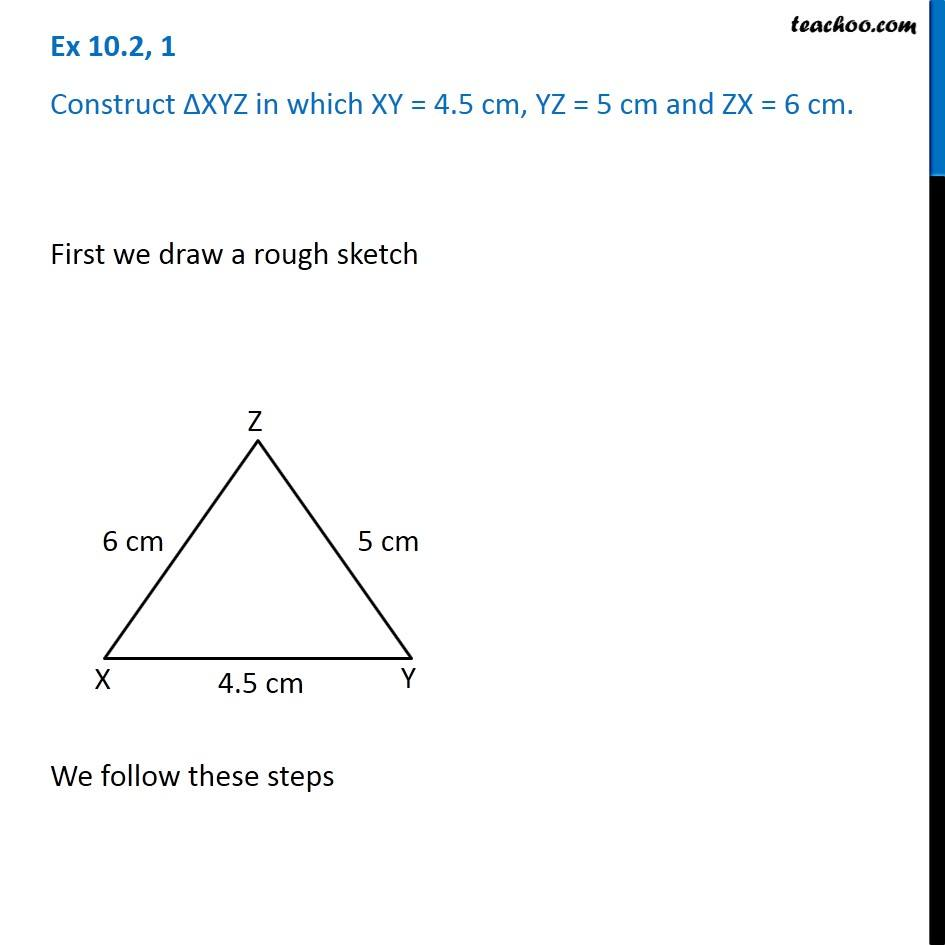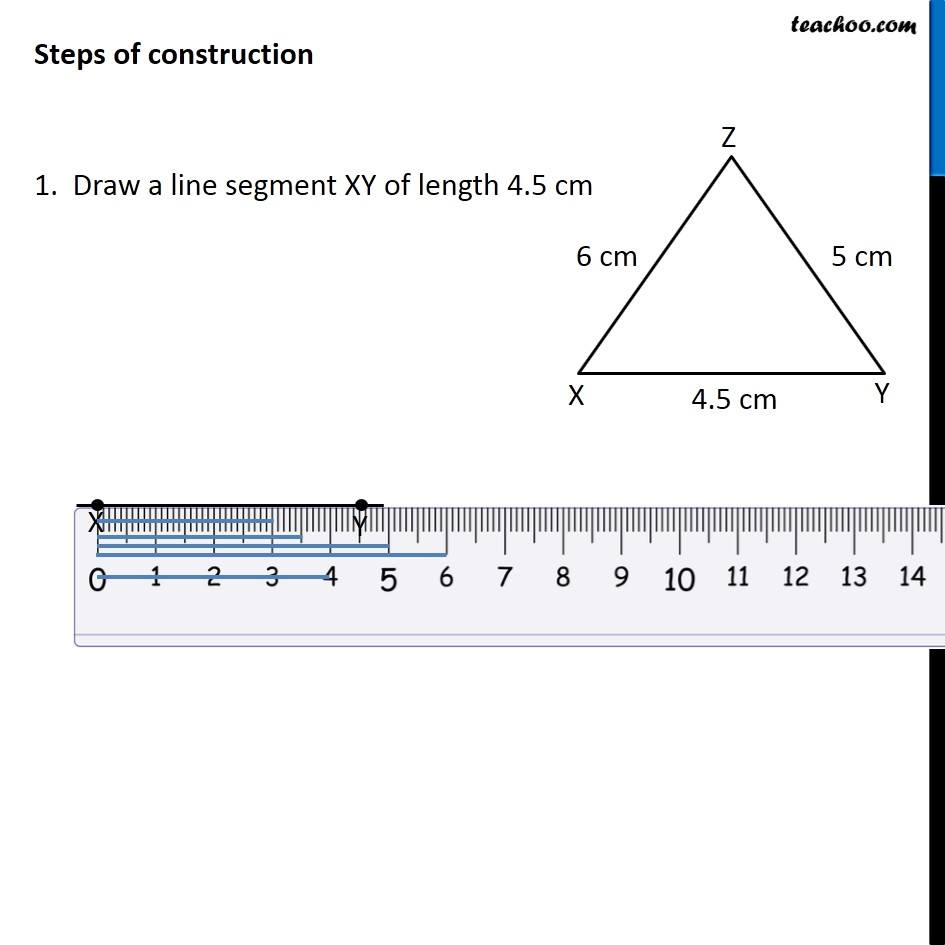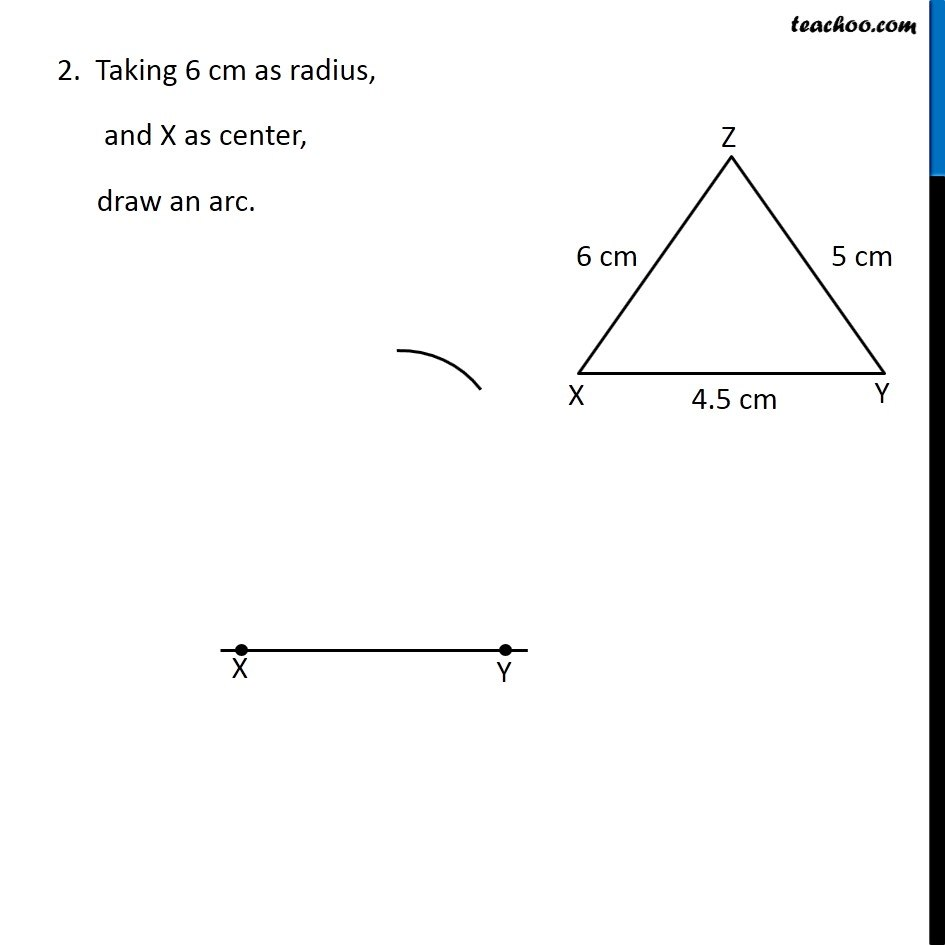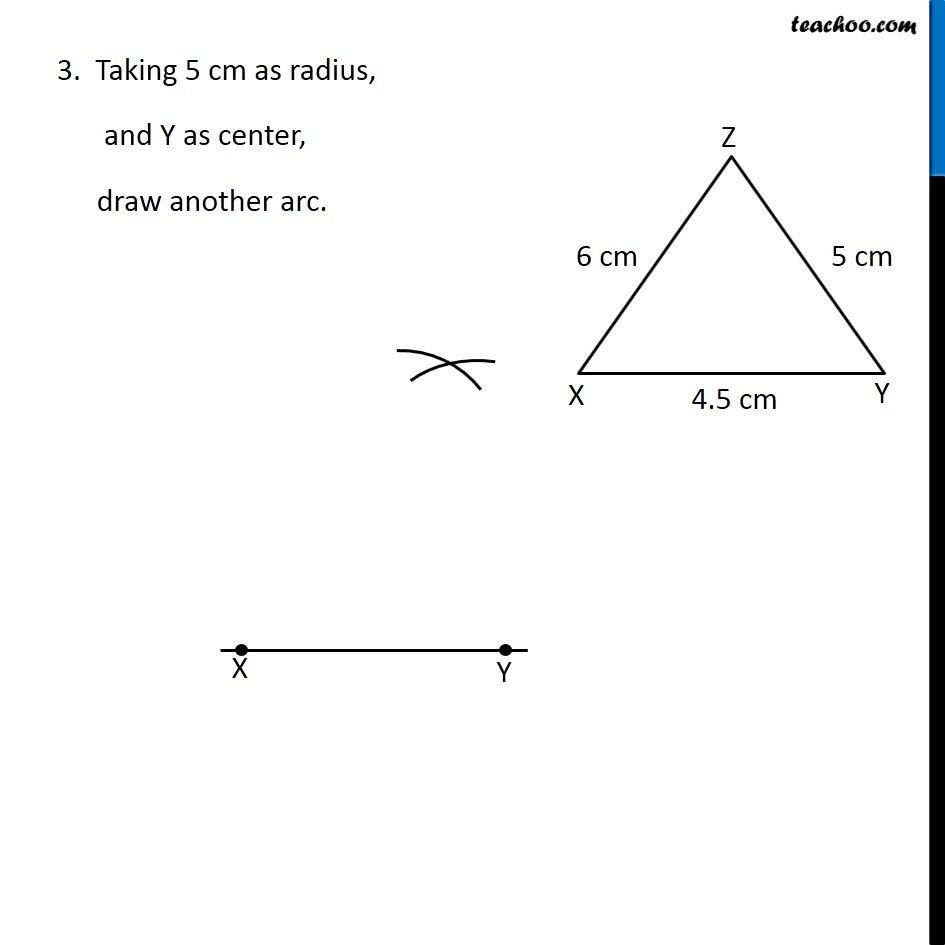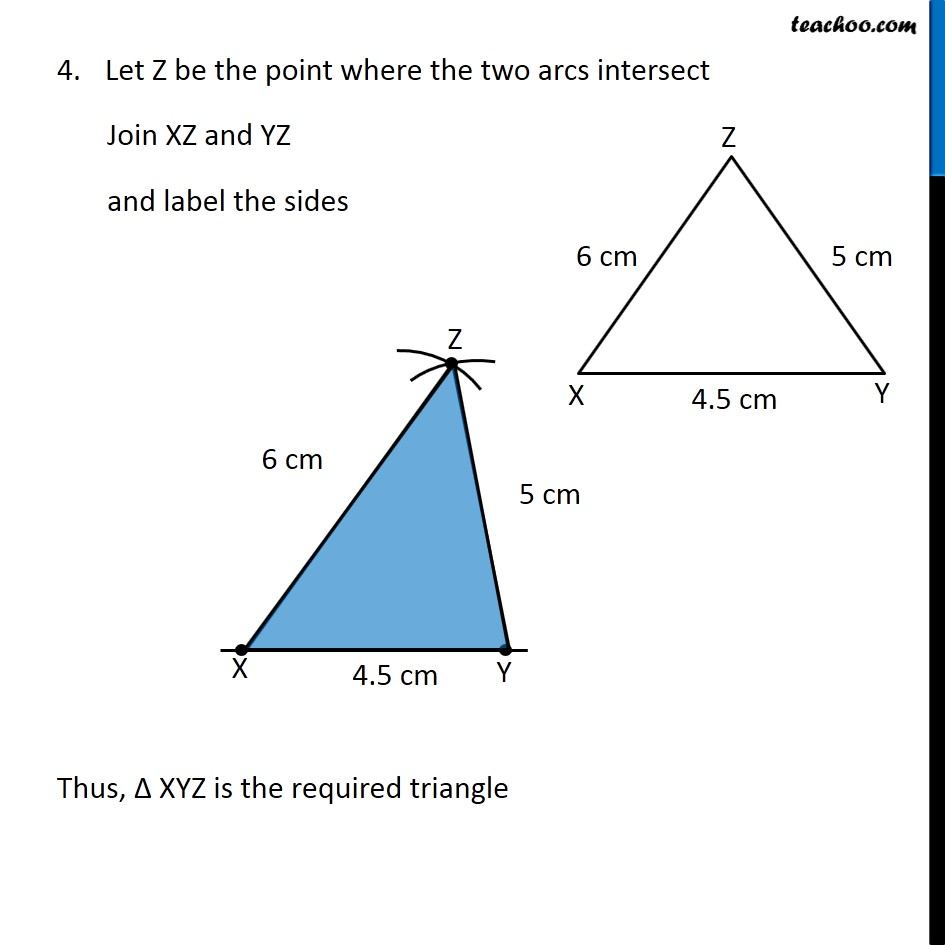1. Chapter 10 Class 7 Practical Geometry
2. Concept wise
3. Constructing a triangle when 3 sides known (SSS)

Transcript

Ex 10.2, 1 Construct ∆XYZ in which XY = 4.5 cm, YZ = 5 cm and ZX = 6 cm. First we draw a rough sketch We follow these steps Steps of construction 1. Draw a line segment XY of length 4.5 cm 2. Taking 6 cm as radius, and X as center, draw an arc. 3. Taking 5 cm as radius, and Y as center, draw another arc. Let Z be the point where the two arcs intersect Join XZ and YZ and label the sides Thus, Δ XYZ is the required triangle

Constructing a triangle when 3 sides known (SSS)